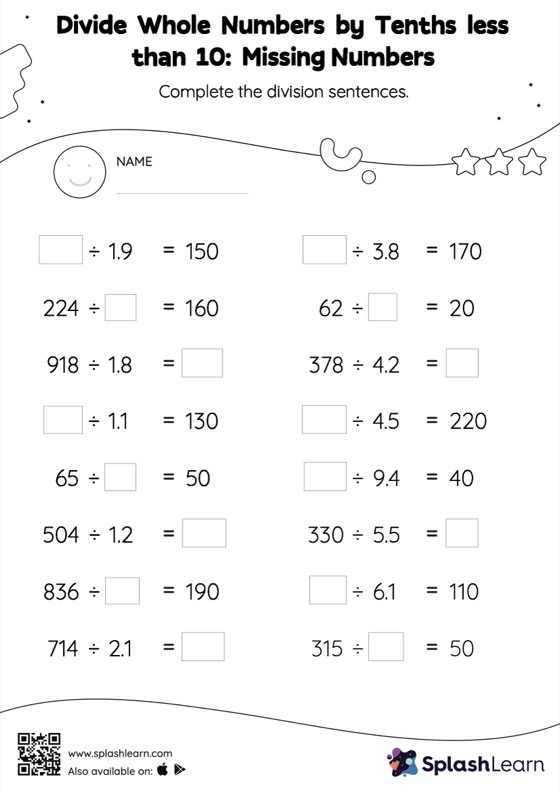# Divide Whole Numbers by Tenths less than 10 without Remainder: Missing Numbers Worksheet

Home > Divide Whole Numbers by Tenths less than 10 without Remainder: Missing NumbersThis worksheet consists of a set of questions on division where students are required to divide whole numbers by tenths less than 10 without remainder. When dividing a number by a decimal, students divide without considering the decimal point and then move each digit of the quotient to the left based on the number of decimal digits in the divisor. Divide whole numbers by tenths less than 10 without remainder provides plenty of practice with this concept as they find the missing number in decimal division. In each problem, the numbers are laid out in the horizontal format. Students should try to use different strategies involving composing and decomposing numbers to solve these problems. This will help them develop flexibility and fluency.